## Separable Equations

Simply put, a differential equation is said to be separable if the variables can be separated. That is, a separable equation is one that can be written in the form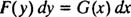Once this is done, all that is needed to solve the equation is to integrate both sides. The method for solving separable equations can therefore be summarized as follows: Separate the variables and integrate.

Example 1: Solve the equation 2 y dy = ( x 2 + 1) dx.

Since this equation is already expressed in “separated” form, just integrate: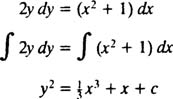Example 2: Solve the equationThis equation is separable, since the variables can be separated:The integral of the left‐hand side of this last equation is simply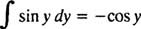and the integral of the right‐hand side is evaluated using integration by parts: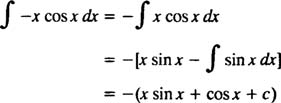The solution of the differential equation is therefore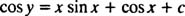Example 3: Solve the IVP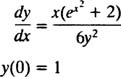The equation can be rewritten as follows:Integrating both sides yields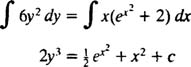Since the initial condition states that y = 1 at x = 0, the parameter c can be evaluated:The solution of the IVP is therefore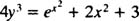Example 4: Find all solutions of the differential equation ( x 2 – 1) y 3 dx + x 2 dy = 0.

Separating the variables and then integrating both sides gives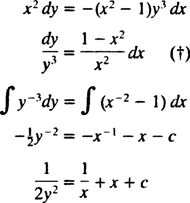Although the problem seems finished, there is another solution of the given differential equation that is not described by the family ½ y −2 = x −1 + x + c. In the separation step marked (†), both sides were divided by y 3. This operation prevented y = 0 from being derived as a solution (since division by zero is forbidden). It just so happens, however, that y = 0 is a solution of the given differential equation, as you can easily check (note: y = 0 ⇒ dy = 0).

Thus, the complete solution of this equation must includeThe lesson is clear:

If both sides of a separable differential equation are divided by some function f( y) (that is, a function of the dependent variable) during the separation process, then a valid solution may be lost. As a final step, you must check whether the constant function y = y 0 [where f( y 0) = 0] is indeed a solution of the given differential equation. If it is, and if the family of solutions found by integrating both sides of the separated equation does not include this constant function, then this additional solution must be separately stated to complete the problem.

Example 5: Solve the equation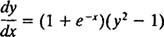Separating the variables gives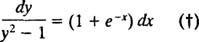(To achieve this separated form, note that both sides of the original equation were divided by y 2 – 1. Thus, the constant functions y = 1 and y = ‐1 may be lost as possible solutions; this will have to be checked later.) Integrating both sides of the separated equation yieldsNow, both constant functions y = 1 and y = –1 are solutions of the original differential equation (as you can check by simply noting that y = ±1 ⟹ dy/ dx = 0), and neither is described by the family above. Thus the complete set of solutions of the given differential equation includesExample 6: Solve the differential equation xydx – ( x 2 + 1) dy = 0.

Separate the variables,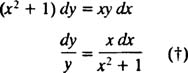and integrate both sides: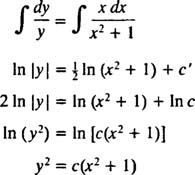Note that in the separation step (†), both sides were divided by y; thus, the solution y = 0 may have been lost. Direct substitution of the constant function y = 0 into the original differential equation shows that it is indeed a solution. However, the family y 2 = c( x 2 + 1) already includes the function y = 0 (take c = 0), so it need not be separately mentioned.

Example 7: Find the curve r = r(θ) in polar coordinates that solves the IVP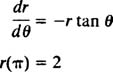The given equation is separable, since it can be expressed in the separated form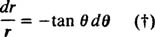Now integrate both sides: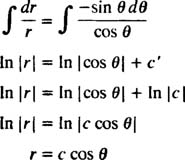Since the solution curve is to pass through the point with polar coordinates ( r, θ) = (2, π),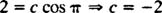The solution of the IVP is therefore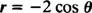This is a circle of diameter 2, tangent to the y axis at the origin; see Figure . Note: In the separation step (†), both sides were divided by r (which is the dependent variable here). However, even though r = 0 formally satisfies the differential equation, it clearly does not satisfy the initial condition r(π) = 2.